# Hypothesis Testing

Hypothesis Testing is the process of verifying if a hypothesis is viable or not. More specifically, we test to determine whether should we reject a hypothesis in favor of the other hypothesis.

The main hypothesis we are testing is called Null Hypothesis (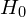), which states that there is no difference in some properties of 2 datasets (each of these 2 datasets can be real or theoretical), while on the opposite, there is an Alternative Hypothesis (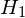) which states that there is a (general or specific) difference between these 2 datasets.

For example, you just get it that for all 50 cities in your country, the average temperature in October is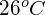, you also know that the temperature of the world this month is normally distributed with the mean of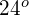C and the standard deviation of 3. Now you want to verify that if your country is significantly different from the average of the world, your hypotheses should be: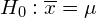. (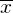is your cities’ mean temperature,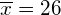.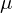is the world’s mean temperature,= 24.)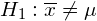.

Since ‘different‘ may mean hotter or colder (higher or lower), this is called the 2-tailed test.

If what you want to verify is whether your country is significantly hotter than the overall, yourwould be changed to: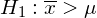.

And this is called a 1-tailed test.

Note thatandmust be mutually exclusive and there is one and only one is true.

After stating the 2 hypotheses, we should define a significance level. Significance level (denoted by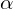) is the probability such that if there is less than this probability for the 2 datasets to exist given the null hypothesis is true, we will reject the null hypothesis and accept the alternative hypothesis. Normally, the significance level has a value of 5%, 2.5% or 1%.

For above example (with), and suppose we choose= 5%. If after examining, we get that: if we take a sample set of size 50 from a normal distribution with mean 24 and std 3, and there is less than 5% that this set’s mean value is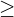26, then we reject the null hypothesis and accept the alternative hypothesis that your country is significantly hotter than the world. If the probability of getting a set of size 50 with a mean value of26 is at least 5%, then we failed to reject the null hypothesis.

About how to compute this probability, we will elaborate in another post. For now, let me tell you the answer: From a normal distribution of mean 24 and std 3, the probability that we can get a set of 50 sample data points with an average value ofis 0.0001%. This is much smaller than 5%, so we reject the null hypothesis and accept the alternative hypothesis.

The above 0.0001% is called the p-value. This term is very important, so remember: p-value is the conditional probability of obtaining an at least as an extreme result as your actual data given the null hypothesis is true. When the p-value is less than the Significance level, we reject the Null hypothesis. (Otherwise, when the p-value is higher than, we failed to reject the Null hypothesis.)

There is another term that is often used to determine the acceptance or rejection of the Null hypothesis, the Critical value. The relationship between Critical value and the score (says, T-score or Z-score) is pretty similar to the significance level and the p-value. The critical value shows how extreme our score should at least be in order to reject the Null hypothesis. So if our (T- or Z-)score isthe critical value, we reject the Null hypothesis, while we accept the Null hypothesis otherwise.

Note that the above 2 relationships are equivalent, meaning there never exists a case when one indicates a rejection when another indicates an acceptance of the Null hypothesis.

Well, there are a lot of terminologies, right? Please bear with me, there are only a few lefts. The next terms are confidence level and confidence interval.

The Confidence Level is expressed in a percentage form (e.g. 90% confidence level, 95% confidence level), while a value of Confidence Interval is a range (or say an interval) like [20, 30] or [-3, 5].

Return to the above example, you have your cities’ mean temperature on October to be, and let’s suppose the std of them is 2, and, by computation, you get that: with 95% confidence level, the true mean temperature of the world on October is in the range 26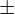0.55C, or [25.45, 26.55]C (the confidence interval).

A 95% confidence level means that: if you repeatedly pick 50 random cities in the world and compute confidence intervals like this, there are 95% of the times that the confidence intervals cover the true population mean.

The value 0.55, which is half the width of the confidence interval, is called the Margin of Error. The smaller the margin of error, the more confidence we have that our actual data is close to the true population.

On a side note, there is something weird here. We state that with a 95% confidence level that the average October’s temperature of the world is in the range [25.45, 26.55]C, while the actual value is 24C, which is very far from the range. The reason for this is that we have a fault in testing our hypothesis. Because cities in your country are close to each other, they share very similar weather properties that define the temperature (e.g. distance to the equator, monsoon, rainfall, hours of daylight), thus they cannot effectively represent the world. Statistically speaking, to use a sample set to extrapolate the population, the sample set must be randomly picked. In this example, all the data points we choose are cities in your country, so the process is not random, which makes a big error in our conclusion.

To sum up

This post gives an introduction to hypothesis testing. The key takeaways we should remember are:

Hypothesis Testing is the process of verifying if a hypothesis is viable or not.

There are 2 hypotheses in the process. The Null hypothesis () states that there is no difference in some properties of 2 datasets, while the Alternative Hypothesis () states that there is a difference between these 2 datasets.

Depending on if the Alternative Hypothesis is general (just stating there is a difference) or specific (states about higher or lower) that the test is called a 2-tailed test or 1-tailed test, respectively.

The p-value is the conditional probability of obtaining an at least as an extreme result as your actual data given the null hypothesis is true.

The critical value shows how large the (T- or Z-)score must be to reject the Null hypothesis.

The Significance level (denoted by) is the probability such that if p-value <, we will reject the null hypothesis and accept the alternative hypothesis.

The Confidence level is expressed in a percentage form, while the value of the confidence interval is a range (an interval). These 2 values are paired with each other. We say something like: with x% confidence level that the confidence interval is [some value, some value]. The 95% confidence level in the given example means that: if you repeatedly pick 50 random cities in the world and compute confidence intervals, there is 95% of the times that the confidence intervals cover the true population mean.

The Margin of Error equals half the width of the confidence interval.

To use a sample set to extrapolate the population, the sample set must be a good representative of the whole population.

References:

## 2 thoughts on “Hypothesis Testing”

1.tdang says:

Thanks for a clear explanation. I’d like to add that one important application of hypothesis testing is to infer information about a sample to population. In real, it’s quite difficult to measure statistical parameters about a whole population, we instead, practically can conduct survey on a sample. From stats of that sample, what can we say about the stats of population.
It would be great if author could give some examples like that. They are likely more convinced than the mentioned one in this post.

Regards,
Truong Dang.

1.Tung.M.Phung says: